Back to the homepage - Zpet na domovskou stranku
Open problems
 Seigneur, ouvre mes lèvres, et ma bouche publiera ta louange.

Psaume 51

## Research Papers and Publications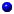Envelopes of open sets and extending holomorphic functions on dual Banach spaces (with D.Garcia and M.Maestre; Preprint no. MATH-KMA-2009/306, see KMA Preprint Series; http://arxiv.org/abs/0905.2531)A note on intersections of simplices (with D.A.Edwards and J.Spurny; Preprint no. MATH-KMA-2009/304, see KMA Preprint Series)The structure of Valdivia compact lines (with W.Kubis; Preprint no. MATH-KMA-2008/284, see KMA Preprint Series)Boundaries of compact convex sets and fragmentability (with J.Spurny; J. Funct. Anal. 256 (2009), no. 3, 865-880; doi:10.1016/j.jfa.2008.11.004; Preprint no. MATH-KMA-2008/262)Natural examples of Valdivia compact spaces (Preprint no. MATH-KMA-2007/247; J. Math. Anal. Appl. 340 (2008), no. 1, 81-101; doi:10.1016/j.jmaa.2007.07.069)Quotients of continuous convex functions on nonreflexive Banach spaces (with P.Holicky, L.Vesely and L.Zajicek; Preprint no. MATH-KMA-2007/241; Bull. Acad. Polon. Sci. 55 (2007), no. 3, 211-217.)On products with the unit interval (Preprint no. MATH-KMA-2007/233; Topol. Appl. 155 (2008), no. 10, 1098-1104; doi:10.1016/j.topol.2008.01.010)Fiber orders and compact spaces of uncountable weight (with A.Aviles; Fundamenta Mathematicae; Preprint no. MATH-KMA-2006/227, see KMA Preprint Series)(I)-envelopes of unit balls and James' characterization of reflexivity (Preprint no. MATH-KMA-2006/202; Studia Math. 182 (2007), no. 1, 29-40.)The convergence space of minimal usco mappings (with R.Anguelov; Preprint no. MATH-KMA-2006/197; Czechoslovak Math. J. 59 (134) (2009), no. 1, 101-128; doi:10.1007/s10587-009-0008-4)Baire-one mappings contained in a usco map (Preprint no. MATH-KMA-2006/196; Comment. Math. Univ. Carolinae 48 (2007), no. 1, 135-145.)(I)-envelopes of closed convex sets in Banach spaces (Preprint no. MATH-KMA-2006/191; Israel J. Math. 162 (2007), no. 1, 157-181.)A converse to Amir-Lindenstrauss theorem in complex Banach spaces (Preprint no. MATH-KMA-2006/190; Rev. R. Acad. Cien. Serie A. Mat. 100 (2006), no. 1-2, 183-190.)Complex Banach spaces with Valdivia dual unit ball ( Extracta Math. 20 (2005), no.3, 243-259; Preprint no. MATH-KMA-2005/187)C(K) spaces which cannot be uniformly embedded into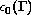(with J.Pelant and P.Holicky, Fundamenta Mathematicae 192 (2006), 245-254; Preprint no. MATH-KMA-2005/174)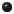Descriptive hierarchy of complex Banach spaces, Spring 2005, unpublished manuscript - pdf file (237 kB); Preprint no. MATH-KMA-2005/169, see KMA Preprint SeriesA solution of the abstract Dirichlet problem for Baire-one functions (with J.Spurny; J. Funct. Anal. 232 (2006), 259-294; Preprint no. MATH-KMA-2005/167)Remark on the abstract Dirichlet problem for Baire-one functions (Preprint no. MATH-KMA-2004/143, Bull. Acad. Polon. Sci. 53 (2005), no. 1, 55-73.)Extending Baire-one functions on topological spaces (with J.Spurny;Topol. Appl. 149 (2005), 195-216; Preprint no. MATH-KMA-2004/133)Note on Countable Unions of Corson Countably Compact Spaces (Preprint No. MATH-KMA-2003/113; Comment. Math. Univ. Carolinae 45 (2004), no. 3, 499-507)Descriptive Properties of Spaces of Signed Measures (with M.Raja; Preprint No. MATH-KMA-2003/109, Acta Univ. Carolinae 44 (2003), no. 2, 79-88)Filling Analytic Sets by the Derivatives of C1-Smooth Bumps (with M.Fabian and J.Kolar; Preprint No. MATH-KMA-2002/76; Proc. Amer. Math. Soc. 133 (2005), no. 1, 295-303)On the Class of Continuous Images of Valdivia Compacta (Preprint No. MATH-KMA-2002/75; Extracta Math. 18 (2003), no.1, 65-80)On Subclasses of Weak Asplund Spaces (with K.Kunen, Preprint No. MATH-KMA-2001/62; Proc. Amer. Math. Soc. 133 (2005), no.2, 425-429)A Weak Asplund Spaces Whose Dual is not in Stegall's Class (Preprint No. MATH-KMA-2001/59, Proc. Amer. Math. Soc. 130 (2002), no. 7, 2139-2143)M-bases in Spaces of Continuous Functions on Ordinals (Preprint No. MATH-KMA-2001/55; Colloquium Math. 92 (2002), no. 2, 179-187)A New Banach Space with Valdivia Dual Unit Ball (Preprint No. MATH-KMA-2001/46; Israel J. Math. 131 (2002), 139-147)Note on Markushevich Bases in Subspaces and Quotients of Banach Spaces (Preprint No. MATH-KMA-2000/39; Bull. Acad. Polon. Sci. 50 (2002), no. 2, 117-126)A Characterization of Weakly Lindelöf Determined Banach Spaces (Preprint No. KMA-2000-29; Serdica Math. J. 29 (2003), no. 2, 95-108)On Double-derived Sets in Topological Spaces (Houston Journal of Mathematics 26 (2000), no. 4, 807-809)Valdivia Compact Spaces in Topology and Banach Space Theory (a survey paper, Extracta Math. 15, no.1, 2000, 1-85)Valdivia Compacta and Biduals of Asplund Spaces (Preprint No. KMA-1999-14; in T.Banakh (ed.), `General Topology in Banach spaces', Nova Sci. Publ., Huntington, New York, 2001, 115-125)Valdivia Compacta and Subspaces of C(K) Spaces (Preprint No. KMA-1999-12; Extracta Math. 14, no. 3(1999), 355-371)Embedding the Ordinal Segment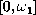into Continuous Images of Valdivia Compacta (Preprint No. KMA-1999-3; Comment. Math. Univ. Carolinae 40, no. 4, (1999), 777-783)Valdivia Compacta and Equivalent Norms (Preprint No. KMA-1999-2; Studia Mathematica 138, no. 2, (2000), 179-191)A Characterization of Valdivia Compact Spaces (Collectanea Mathematica 51, no. 1,(2000), 59-81)Continuous Images and Other Topological Properties of Valdivia Compacta ( Fundamenta Mathematicae 162 (1999), no.2, 181-192)An Example Concerning Valdivia Compact Spaces ( Serdica Math. J., 25 (2) (1999), 131-140)Descriptive Properties of Spaces of Measures (with P.Holicky, Bull. Acad. Polon. Sci., 47 (1) (1999), 37-51)Weakly Stegall spaces, Spring 1997, unpublished manuscript - pdf file (99 kB)Stegall Compact Spaces which are not Fragmentable (Topol. Appl., 96 (2) (1999), 121-132)New Examples of Hereditarily t-Baire Spaces ( Bull. Acad. Polon. Sci., 46 (2) (1998), 197-210)Note on Connections of the Point of Continuity Property and Kuratowski Problem on Function Having the Baire Property (Acta Univ. Carolinae, 38 (1) (1997), 3-12)Remark on the Point of Continuity Property II (with P.Holicky, Bull. Acad. Polon. Sci., 43 (2) (1995), 105-111)

## ThesesClasses of compact spaces in functional analysis (Habilitation Thesis, MFF UK, 2004)

Contents:
• Preface
• Introduction
• Valdivia compacta and continuous images
• Markushevich bases and primarily Lindelöf spaces
• Valdivia type Banach spaces
• Bibliography
Preface, Introduction, the introductory notes to the remaining chapters and Bibliography are contained in this pdf file (297 kB). Except for this the thesis contains six published papers from the above list.Continuity-like Properties of Mappings (PhD Thesis, MFF UK 1997)
Contents:
• Introduction - pdf file (58 kB)
• Remark on the Point of Continuity Property II (a published joint paper with P.Holicky, see above)
• Note on Connections of Point of Continuity Property and Kuratowski Problem on Function Having the Baire Property (a published paper, see above)
• New Examples of Hereditarily t-Baire Spaces (a published paper, see above)
• Stegall Compact Spaces Which Are Not Fragmentable (a published paper, see above)
• Few remarks on structure of certain spaces of measures - pdf file (209 kB)Dedicne Baireovy prostory a vlastnost bodu spojitosti (Hereditarily Baire Spaces and Point of Continuity Property, in Czech) (Diplomova prace - Master Thesis, MFF UK 1995, pdf file (429 kB))

## Abstracta of the Above Papers

• Envelopes of open sets and extending holomorphic functions on dual Banach spaces
We investigate certain envelopes of open sets in dual Banach spaces which are related to extending holomorphic functions. We give a variety of examples of absolutely convex sets showing that the extension is in many cases not possible. We also establish connections to the study of iterated weak* sequential closures of convex sets in the duals of separable spaces.

• A note on intersections of simplices
We provide a corrected proof of Theorem 9 in [D.A. Edwards, Systèmes projectifs d'ensembles convexes compacts, Bulletin de la S.M.F. 103 (1975), 225-240] stating that any metrizable infinite-dimensional simplex is affinely homeomorphic to the intersection of a decreasing sequence of Bauer simplices.

• The structure of Valdivia compact lines
We study linearly ordered spaces which are Valdivia compact in their order topology. We find an internal characterization of these spaces and we present a counterexample disproving a conjecture posed earlier by the first author. The conjecture asserted that a compact line is Valdivia compact if its weight does not exceed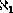, every point of uncountable character is isolated from one side and every closed first countable subspace is metrizable. It turns out that the last condition is not sufficient. On the other hand, we show that the conjecture is valid if the closure of the set of points of uncountable character is scattered. This improves an earlier result of the first author.

• Boundaries of compact convex sets and fragmentability
If X is a compact convex set in a real locally convex space, a subset B of X is said to be its boundary if every affine continuous function on X attains its maximum at some point of B. We study relations between fragmentability of B and the whole set X and a connection of this question to the boundary problem. As a byproduct we obtain a characterization of separable Asplund spaces. We further prove the positive answer to the boundary problem under some topological assumptions on the boundary. We also study the possibility of finding the Haar system in a boundary of a metrizable compact convex set.

• Natural examples of Valdivia compact spaces
We collect examples of Valdivia compact spaces, their continuous images and associated classes of Banach spaces which appear naturally in various branches of mathematics. We focus on topological constructions generating Valdivia compact spaces, linearly ordered compact spaces, compact groups, L1 spaces, Banach lattices and non-commutative L1 spaces.

• Quotients of continuous convex functions on nonreflexive Banach spaces
On each nonreflexive Banach space X there exists a positive continuous convex function f such that 1/f is not a d.c. function (i.e., a difference of two continuous convex functions). This result together with known ones implies that X is reflexive if and only if each everywhere defined quotient of two continuous convex functions is a d.c. function. Our construction gives also a stronger version of Klee's result concerning renormings of nonreflexive spaces and non-norm-attaining functionals.

• On products with the unit interval
We investigate the question which compact convex sets are homeomorphic to their product with the unit interval. We prove it in particular for the space of probability measures on any infinite scattered compact space and for the half-ball of a nonseparable Hilbert space equipped with the weak topology. We also show examples of compact spaces for which it is not the case.

• Fiber orders and compact spaces of uncountable weight
We study an order relation on the fibers of a continuous map and its application to the study of the structure of compact spaces of uncountable weight.

• (I)-envelopes of unit balls and James' characterization of reflexivity
We study the (I)-envelopes of the unit balls of Banach spaces. We show, in particular, that any nonreflexive space can be renormed such that the (I)-envelope of the unit ball is not the whole bidual unit ball. Further, we give a simpler proof of the James' characterization of reflexivity in nonseparable case. We also study the spaces in which the (I)-envelope of the unit ball adds nothing.

• The convergence space of minimal usco mappings
A convergence structure generalizing the order convergence structure on the set of Hausdorff continuous interval functions is defined on the set of minimal usco maps. The properties of the obtained convergence space are investigated and essential links with the pointwise convergence and the order convergence are revealed. The convergence structure can be extended to a uniform convergence structure so that the convergence space is complete. The important issue of the denseness of the subset of all continuous functions is also addressed.

• Baire-one mappings contained in a usco map
We investigate Baire-one functions whose graph is contained in a graph of usco mapping. We prove in particular that such a function defined on a metric space with values in Rd is the pointwise limit of a sequence of continuous functions with graphs contained in the graph of a common usco map.

• (I)-envelopes of closed convex sets in Banach spaces
We study the notion of (I)-generating introduced by V. Fonf and J. Lindendstrauss and a related notion of (I)-envelope. As a consequence of our results we get an easy proof of the James characterization of weak compactness in Banach spaces with weak* angelic dual unit ball and an easy proof of the James characterization of reflexivity within a large class of spaces. We also show by an example that the general James theorem cannot be proved by this method.

• A converse to Amir-Lindenstrauss theorem in complex Banach spaces
We show that a complex Banach space is weakly Lindelöf determined if and only if the dual unit ball of any equivalent norm is weak* Valdivia compactum. We deduce that a complex Banach space X is weakly Lindelöf determined if and only if any nonseparable Banach space isomorphic to a complemented subspace of X admits a projectional resolution of the identity. These results complete the previous ones on real spaces.

• Complex Banach spaces with Valdivia dual unit ball
We study the classes of complex Banach spaces with Valdivia dual unit ball. We give complex analogues of several theorems of real spaces. Further we study relationship of these complex Banach spaces with their real versions and that of real Banach spaces and their complexification. We also formulate several open problems.

• C(K) spaces which cannot be uniformly embedded intoWe give two examples of scattered compact spaces K such that C(K) is not uniformly homeomorphic to any subset offor any set. The first one isand hence it has the smallest possible cardinality, the other one has the smallest possible height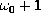.
(Foreword:We present two results of Jan Pelant. He presented them at seminars in the Mathematical Institute of Czech Academy of Sciences during last two years. The example described in Theorem 4.1 below is quite recent. Unfortunately, Jan Pelant died before the results were prepared for publication. We decided to reconstruct them using his hand-written notes. )

• A solution of the abstract Dirichlet problem for Baire-one functions
Let X be a compact convex subset of a locally convex space. We show that any bounded Baire-one function defined on ext X can be extended to an affine Baire-one function on X if and only if X is a Choquet simplex and ext X satisfies a certain topological property.

• Remark on the abstract Dirichlet problem for Baire-one functions
We study the possibility of extending any bounded Baire-one function on the set of extreme points of a compact convex set to an affine Baire-one function and related questions. We give complete solutions to these questions within a class of Choquet simplicies introduced by P.J.Stacey (1979). In particular we get an example of a Choquet simplex such that its set of extreme points is not Borel but any bounded Baire-one function on the set of extreme points can be extended to an affine Baire-one function. We also study the analogous questions for functions of higher Baire classes.

• Extending Baire-one functions on topological spaces
We investigate the possibility of extension of Baire-one functions from subspaces of topological spaces. In particular we prove that any Baire-one function on a Lindelöf hereditarily Baire completely regular space can be extended to a Baire-one function on any completely regular superspace.

• Note on Countable Unions of Corson Countably Compact Spaces
We show that a compact space K has a dense set of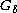points if it can be covered by countably many Corson countably compact spaces. If these Corson countably compact spaces may be chosen to be dense in K, then K is even Corson.

• Descriptive Properties of Spaces of Signed Measures
Topological spaces of signed Radon measures on a Tychonoff space X inherit some descriptive properties of the space X. We show in particular that the space of signed Radon measures on an absolute Borel (Cech-analytic) space is again absolute Borel (Cech-analytic). Main tool in proving such results is study of measurability of evaluation functions.

• Filling analytic sets by the derivatives of C1-smooth bumps
If X is an infinite-dimensional Banach space, with separable dual, and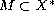is an analytic set such that any pointcan be reached from 0 by a continuous path contained (except for the point x*) in the interior of M, then M is the range of the derivative of a C1-smooth function on X with bounded nonempty support.

• On the Class of Continuous Images of Valdivia Compacta
We study properties of the class of continuous images of Valdivia compact spaces. We introduce its subclass (weakly Corson compact spaces) which is closed to taking subspaces. Further we investigate Banach spaces with dual unit ball being a continuous image of a Valdivia compactum or weakly Corson and establish several stability and non-stability properties.

• On Subclasses of Weak Asplund Spaces
Assuming the consistency of the existence of a measurable cardinal, it is consistent to have two Banach spaces, X,Y, where X is a weak Asplund space such that X* (in the weak* topology) in not in Stegall's class, whereas Y* is in Stegall's class but is not weak* fragmentable.

• A Weak Asplund Spaces Whose Dual is not in Stegall's Class
We show that, under some additional set-theoretical assumptions which are equiconsistent with the existence of a measurable cardinal, there is a weak Asplund space whose dual equipped with the weak* topology is not in the Stegall class. This completes a result by Kenderov, Moors and Sciffer.

• M-bases in Spaces of Continuous Functions on Ordinals
We prove in particular that the spacehas no countably norming Markushevich basis. This answers a question asked by G.Alexandrov and A.Plichko.

• A New Banach Space with Valdivia dual unit ball
We give an example of a Banach space which admits no projectional resolution of the identity but whose dual unit ball in weak* topology is a Valdivia compact. This answers a question asked by M.Fabian, G.Godefroy and V.Zizler.

• Note on Markushevich Bases in Subspaces and Quotients of Banach Spaces
Let us call a Banach space Plichko (1-Plichko) if it admits a countably norming (countably 1-norming) Markushevich basis. We introduce a subclass of Plichko spaces strictly containing weakly Lindelöf determined spaces which is stable to isomorphisms, subspaces and quotients. Further we prove that each quotient of the spaceis 1-Plichko.

• A Characterization of Weakly Lindelöf Determined Banach Spaces
We prove that a Banach space X is weakly Lindelöf determined if (and only if) each non-separable Banach space isomorphic to a complemented subspace of X has a projectional resolution of the identity. This answers a question posed by S.Mercourakis and S.Negrepontis and yields a converse of Amir-Lindenstrauss'theorem. We also prove that a Banach space of the form C(K)where K is a continuous image of a Valdivia compactum is weakly Lindelöf determined if (and only if) each non-separable Banach space isometric to a subspace of C(K) has a projectional resolution of the identity.

• On Double-derived Sets in Topological Spaces
We characterize topological spaces which have a subset with non-closed double-derived set. As a corollary we obtain that the double-derived set of an arbitrary subset of a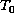topological space is closed. This answers in the negative a question asked by A.Lelek in Houston Problem Book (1995).

• Valdivia Compacta and Biduals of Asplund Spaces
We give an example of an Asplund space -with an equivalent norm - whose bidual unit ball is not a Valdivia compact. This answers in the negative a question of J.Orihuela (1992). Further we introduce a subclass of Asplund spaces, which has nice stability properties and each element of which has Valdivia bidual unit ball. This class contains all Asplund spaces whose dual unit ball is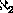-Corson (in particular, all Asplund spaces of densityand all Asplund weakly compactly generated spaces).

• Valdivia Compacta and Subspaces of C(K) Spaces
We prove in particular that the dual unit ball of a Banach space X is a Corson compact provided X is of the form C(K) where K is a continuous image of a Valdivia compact space, and the dual unit ball of every subspace of X is Valdivia compact. Another result is that, if K is a non-Corson continuous image of a Valdivia compact, there is a continuous image L ofK such that the dual unit ball of C(L) is not Valdivia.

• Embedding the Ordinal Segmentinto Continuous Images of Valdivia Compacta
We prove in particular that a continuous image of a Valdivia compact space is Corson provided it contains no homeomorphic copy of the ordinal segment. This generalizes a result of R.Deville and G.Godefroy who proved it for Valdivia compact spaces. We give also a refinement of their result which yields a pointwise version of retractions on a Valdivia compact space.

• Valdivia Compacta and Equivalent Norms
We prove that the dual unit ball of a Banach space Xis a Corson compact provided that the dual unit ball with respect to every equivalent norm on X is a Valdivia compact. As a corollary we get that the dual unit ball of a Banach space X of densityis Corson if X has projectional resolution of the identity with respect to every equivalent norm. These results answer questions posed by M.Fabian, G.Godefroy and V.Zizler.

• A Characterization of Valdivia Compact Spaces
We characterize Valdivia compact spaces K in terms of C(K) endowed with a topology introduced by M.Valdivia (1991). This generalizes R.Pol's characterization of Corson compact spaces. Further we study duality, products and open continuous images of Valdivia compact spaces. We prove in particular that the dual unit ball of C(K) is Valdivia wheneverK is Valdivia and that the converse holds whenever K has a dense set ofpoints. Another result is that any open continuous image of a Valdivia compact space with a dense set ofpoints is again Valdivia.

• Continuous Images and Other Topological Properties of Valdivia Compacta
We study topological properties of Valdivia compact spaces. We prove in particular that a compact Hausdorff space K is Corson provided each continuous image of K is a Valdivia compact. This answers a question of M.Valdivia (1997). Another results are that the class of Valdivia compacta is stable with respect to arbitrary products and a generalization of the fact that Corson compacta are angelic.

• An Example Concerning Valdivia Compact Spaces
We prove that the dual unit ball of the space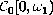endowed with the weak* topology is not a Valdivia compact. This answers a question posed to the author by V.Zizler and has several consequences. Namely, it yields an example of an affine continuous image of a convex Valdivia compact (in the weak* topology of a dual Banach space) which is not Valdivia, and shows that the property of the dual unit ball being Valdivia is not an isomorphic property. Another consequence is that the spacehas no countably 1-norming Markusevic basis.

• Descriptive Properties of Spaces of Measures
The spaces of Borel probabilities on a topological space X inherit a number of topological properties of X. We show in particular that the space of tight probabilities on a Cech-analytic space is Cech-analytic. Analogical results are shown for several other classes of generalized analytic and complete topological spaces.

• Stegall Compact Spaces which are not Fragmentable
Using modifications of the well-known construction of "double-arrow" space we give consistent examples of nonfragmentable compact Hausdorff spaces which belong to Stegall's class $S$. Namely the following is proved.
(1) Ifis less than the least inaccessible cardinal in $L$ andhold then there is a nonfragmentable compact Hausdorff space K such that every minimal usco mapping of a Baire space intoK is singlevalued at points of a residual set.
(2) If V=L then there is a nonfragmentable compact Hausdorff space K such that every minimal usco mapping of a completely regular Baire space intoK is singlevalued at points of a residual set.

• New Examples of Hereditarily t-Baire Spaces
We introduce a new class of hereditarily t-Baire spaces (defined by G.Koumoullis (1993)) which need not to have the restricted Baire property in a compactification - as an example serves the spacefor A uncountable. We use this and a modification of a construction of D.Fremlin (1987) to get, under the assumption that there is a measurable cardinal, an example of a first class function of a hereditarily t-Baire space into a metric space which has no point of continuity, which shows, in answer to a question of G.Koumoullis (1993), that the cardinality restriction in his Theorem 4.1 cannot be dropped.

• Note on Connections of the Point of Continuity Property and Kuratowski Problem on Function Having the Baire Property
It is shown in particular that the question whether every extended Borel class one (e.g.-measurable) map of any hereditarily Baire space into a metric space has the point of continuity property is equivalent to the Kuratowski question whether the function with the Baire property of any topological space into a metric space is continuous apart from a meager set. The method of the proof enables us to get, under the assumption that it is consistent to suppose that there is a measurable cardinal, examples of ordinary Borel class one maps (i.e.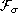-measurable) of a hereditarily Baire space intoa metric space which have not the point of continuity property.These examples complete and strengthen an example of G.Koumoullis, who constructed (under the assumption that there is a real-valued measurable cardinal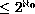an extended Borel class one function (even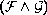-measurable) of a hereditarily Baire space into a metric space with no continuity point but it is not clear whether this map is-measurable.

• Remark on the Point of Continuity Property II
We prove in particular that if X is a hereditarily Baire space which has the tightness less than the least weakly inaccessible cardinal and each (closed) subspace of Xhas the countable chain condition, then every Borel class one map of X into a metric space M has the point of continuity property. In the case of countable tightness the assumption that every closed subspace has the countable chain condition is not needed and we get a result of R.W.Hansell (1991).CESKY - ISO 8859